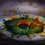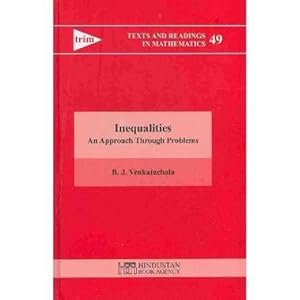# RMO Paper 2013 of Maharashtra and Goa region

$\color{#D61F06}{\text{This year's (2013) RMO paper of Maharashtra and Goa Region .}}$

(1) Find all positive integers $m$ such that $(m-1)!$ is divisible by $m$.

(2) $H$ is the orthocentre of $\triangle ABC$, $D$ is any point on $BC$ if a circle described with centre $D$ and radius $DH$ meets $AH$ produced in $E$, Prove that $E$ lies on the circumcircle of $\triangle ABC$.

(3) Suppose $\triangle ABC$ is an acute angled triangle with $AB. Let $M$ be the midpoint of $BC$. Suppose $P$ is a point on side $AB$ such that, if $PC$ intersects the median $AM$ at $E$, then $AP=PE$.Prove $AB=CE$.

(4) Let $x$ and y be real numbers such that $x^2 + y^2 = 1$

Prove that $\dfrac {1}{x^2+1} +\dfrac {1}{y^2+1}+\dfrac {1}{1+xy} \geq \dfrac {3} {1+\frac {(x+y)^2}{4}}$

(5) Let $a_n$ be number of sequences of n terms formed using the digits $0,1,2$ and $3$ in which $0$ occurs an odd number of times. Find $a_n$

(6) Find all positive integers $n$ such that the product of all the positive divisors of $n$ is equal to $n^3$.7 years, 8 months ago

This discussion board is a place to discuss our Daily Challenges and the math and science related to those challenges. Explanations are more than just a solution — they should explain the steps and thinking strategies that you used to obtain the solution. Comments should further the discussion of math and science.

When posting on Brilliant:

• Use the emojis to react to an explanation, whether you're congratulating a job well done , or just really confused .
• Ask specific questions about the challenge or the steps in somebody's explanation. Well-posed questions can add a lot to the discussion, but posting "I don't understand!" doesn't help anyone.
• Try to contribute something new to the discussion, whether it is an extension, generalization or other idea related to the challenge.

MarkdownAppears as
*italics* or _italics_ italics
**bold** or __bold__ bold
- bulleted- list
• bulleted
• list
1. numbered2. list
1. numbered
2. list
Note: you must add a full line of space before and after lists for them to show up correctly
paragraph 1paragraph 2

paragraph 1

paragraph 2

[example link](https://brilliant.org)example link
> This is a quote
This is a quote
    # I indented these lines
# 4 spaces, and now they show
# up as a code block.

print "hello world"
# I indented these lines
# 4 spaces, and now they show
# up as a code block.

print "hello world"
MathAppears as
Remember to wrap math in $$ ... $$ or $ ... $ to ensure proper formatting.
2 \times 3 $2 \times 3$
2^{34} $2^{34}$
a_{i-1} $a_{i-1}$
\frac{2}{3} $\frac{2}{3}$
\sqrt{2} $\sqrt{2}$
\sum_{i=1}^3 $\sum_{i=1}^3$
\sin \theta $\sin \theta$
\boxed{123} $\boxed{123}$

Sort by:

Find all positive integers n such that the product of all the positive divisors of n is equal to n^3

- 7 years, 7 months ago

Simply find "n" who have 6 positive divisors(including n itself) thus giving 3 pairs that give product exactly $n$ so that product of all divisors being $n^3$........ hence $n$=$p^2_1.p_2$ OR n=$p^5$ OR 1

- 7 years, 7 months ago

How come you have put more than 5 tags?@Aditya Raut

- 6 years, 7 months ago

This discussion was posted before January 2014, so at that time you could add as many tags as you want...

- 6 years, 7 months ago

Oh, btw congrats for clearing RMO. @Aditya Raut

- 6 years, 7 months ago

The third question is very simple one. One needs to use the result that when two cevians intersect the sides of triangles in the ratio m and n respectively, then they divide each other in the ratio m(n+1)/n and n(m+1)/m. Then the question becomes very simple.

- 7 years, 8 months ago

Yes and that formula can be proved simply by Using B.P.T. or Basic Proportionality Theorem...

- 7 years, 8 months ago

which books did you use for RMO? @Aditya Raut

- 5 years, 11 months ago

PlZ give this ques Sol. Actually I am not able to solve this

- 3 years, 10 months ago

- 1 year, 12 months ago

I solved this one using B.P.T and similar triangles.

- 7 years, 8 months ago

$m \in \mathbb{N}-$ {4,p}

where $p$ is prime. (All prime numbers are discarded)

- 7 years, 8 months ago

To cancel all the primes, We can use Wilson's Theorem.

- 7 years, 8 months ago

how did we solve question number 4

- 5 years, 7 months ago

All n such that n=$p^2_1\times p_2$ OR n=$p^5$ OR 1

where p denotes prime.........

- 7 years, 8 months ago

Answer to the 1st one : (m - 1)! is divisible for all positive integers except 4 and primes.

Answer to the 6th one : If you consider n and 1 to be the divisors too, the answers are p^5 and p^2 * q , p and q are primes.

Answer to the 4th one: Simplify the L.H.S and R.H.S of the inequality, such that both the sides contain only xy. After that, using the fact that ( x -y )^2 is always greater than or equal to zero, find out the max value of xy. Plug the max value of xy in the inequality.

- 7 years, 8 months ago

I used Cauchy's inequality to get the results.

- 7 years, 8 months ago

I used Titu's Lemma

- 7 years, 8 months ago

Yeah....we got $xy \leq \frac {1}{2}$ by A.M- G.M inequality to numbers $x^2 , y^2$

- 7 years, 8 months ago

6th problem also has 1as it's solution because positive divisor of 1 is 1 which satisfies product of divisors = $1^3$

- 7 years, 8 months ago

1 and 6 have answers there are infinite solutions

- 3 years, 10 months ago

i will try

- 7 years, 8 months ago

question no. 5 is also very easy.

- 7 years, 8 months ago

I'm selected for the second level. Are you ???

- 7 years, 6 months ago

Why's this incomplete? @Aditya Raut -Care to complete it?

- 7 years ago

DONE .... $\color{#D61F06}{T} \color{#EC7300}{H} \color{limegreen}{A} \color{#20A900}{N} \color{#3D99F6}{K} \color{#69047E}{S} \color{#E81990}{S} \color{darkred}{S}$ .... If you hadn't commented, i wouldn't have known that this paper was later made into half paper.... that might be a mistake by some staff most probably...

- 7 years ago

Thanks a ton!!!!! :)

- 7 years ago

Hi Again! @Aditya Raut -I guess I'd be appearing for RMO dis'yr (That depends on my pre-RMO performance though :P). I;d like to have some tips and prep-method from you. I know that the MG-RMO is much different and easy compared to TN-RMO( which is why we have an equally tough Pre-RMO :P ). ..but yet...You're too good in math...so....even book references would be much appreciated. And-another thing- could you tell me a good resource to learn parametric substitution to solve algebraic equations? I recently noticed this in some problem on this site- and found it fun...I want to know more...Thanks :)

- 7 years ago

Very good, about your learning parametric doubt first, you can try it out on the practice section of brilliant! Or just google it and see all details on the wolfram alpha forum type of website.

If you don't mid give me your email ID plz

And when you reply to this, please mention me. (Hope you'll reply to say thanks :P LOL)

For RMO tips, I recommend you to do what Dinesh said in the discussion of this problem , because me and Dinesh are classmates and I have already said that do what he said (though he didn't clear RMO with me last year, he has double potential to do that).

Book references that I have been using are $\color{#D61F06}{\textbf{Inequalities- An Approach Through Problems}}$ (author B. J. Venkatachala),Inequalities

$\color{#D61F06}{\textbf{Applied Combinatorics}}$ (author Alan Tucker)App. Comb

$\color{#D61F06}{\textbf{Elementary Number Theory}}$ (author David M. Burton)nt

$\color{#D61F06}{\textbf{Problem Solving Strategies}}$ (author Arthur Engel)PSS

$\color{#D61F06}{\textbf{An Excursion in Mathematics}}$ (by Bhaskaracharya Pratishthana, Pune)

$\color{#20A900}{\text{This one i got from Bhaskaracharya Pratishthana for clearing RMO}}$ $\color{#20A900}{\text{(The co-oridinator of RMO in Maharashtra and Goa region)}}$

$\color{#D61F06}{\textbf{IMO problems}}$ (author Istvan Reiman)123

Try previous year RMO papers, that is the most useful thing. Don't look at solutions till you have tried it a lot. And most important, keep calm, because in RMO, every question is solvable, but the $\color{#3D99F6}{\textbf{TRICK}}$ doesn't $\color{#3D99F6}{\textbf{CLICK}}$ at the time, so think on small things.

That's all i can tell, if you want to know more books, or have all the above books for free

(To get these books, I paid ₹ $3379/-$ , but you can get them for free)

Just go to libgen.org or go to bookzz.org and search the book, i am sure you'll get for free. (I downloaded a very costly book named "Learn Python the hard way" for free from here).

- 7 years ago

bro thanks for giving such a useful website

- 6 years, 11 months ago

Anytime ! Wanna be friends ? Gmail- $\color{#3D99F6}{\text{adityaraut34@gmail.com }}$img

- 6 years, 11 months ago

Wow...Thanks A lot for replying @Aditya Raut . I shall try to see the parametric stuff related things on net. Thx again! :) Shall I send you a mail? I guess I saw it in the sol of a problem?

- 7 years ago

Anytime, I am there for anyone who wants to be friends! By the way, please send me email if you want, at $\color{#3D99F6}{\textbf{adityaraut34@gmail.com}}$ instead of 'adityaraut34@yahoo.in', because my yahoo account is related to brilliant and so it is stuffed with emails of "someone liked/reshared/followed" , so for our communication, i prefer GMAIL. Same Applies to my new friend @Satvik Golechha too, please note this.

- 7 years ago

Sure..thanks for notifying this too :P :D

- 7 years ago

please give me the proof of weierstrauss product inequality

- 6 years, 8 months ago

- 6 years, 7 months ago

what is the jensens inequality?

- 6 years, 2 months ago

What is the answer to question 5?

- 5 years, 8 months ago

Is my answer to question 5 correct? $a_n = \frac{3^{n+1}-3}{8}$ if $n$ is even and $a_n = \frac{3^n-1}{8}$ if $n$ is odd?

By the way, I have a few more questions. Was this the same paper for Pune region? Is Arthur Engel's book good?

- 4 years, 11 months ago

@Aditya Raut How to solve the 4 th question can you tell

- 4 years, 5 months ago

pls explain how to solve the 4th one

- 3 years, 10 months ago

You can use cauchy and (x-y)^2 greater than or equal to 0 for 4th question

- 1 year, 9 months ago

The third one is actually quite simple.......... Construct a line parallel to BC from P. Equate the ratio of AP and AB to the ratio of PE and EC ( This can be done by using B.P.T and similar triangles. )

- 7 years, 8 months ago

How to solve the 2nd question?

- 7 years, 7 months ago

where can I find answer key

- 6 years, 8 months ago